# Lines and Angles Class 7 Notes: Chapter 5

## Introduction to Geometry

#### For more information on Introduction to Geometry, watch the below video.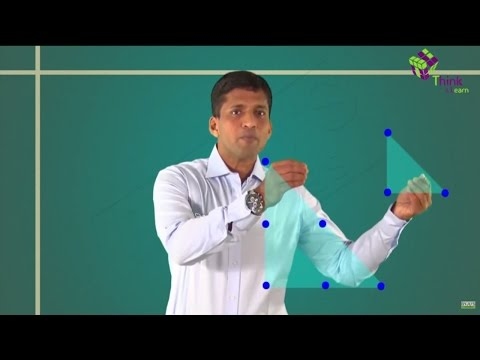To know more about Basics of Geometry, visit here.

### Line, line segment and ray

• If we take a point and draw a straight path that extends endlessly on both the sides, then the straight path is called as a line.
• A ray is a part of a line with one endpoint.
• A line segment is a part of a line with two endpoints.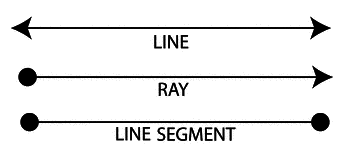To know more about Line Segment and Ray, visit here.

### Angles

• An angle is formed when two rays originate from the same end point.
• The rays making an angle are called the arms of the angle.
• The end point is called the vertex of the angle.
•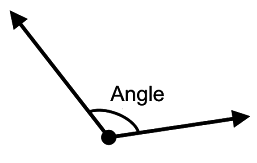To know more about Angles, visit here.

### Complementary Angles

• Two angles whose sum is 900 are called complementary angles.
Example: 50+ 40= 900
∴ 500 and 400 angles are complementary angles.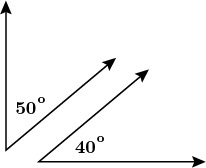To know more about Complementary Angles, visit here.

## Parallel Lines and a Transversal

### Transversal intersecting two lines

• Transversal is a line that intersects two or more lines at different points.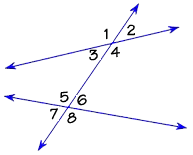• Corresponding Angles:
(i) ∠1 and ∠5 (ii) ∠2 and ∠6
(iii) ∠3 and ∠7 (iv) ∠4 and ∠8
• Alternate Interior Angles:
(i) ∠3 and ∠6 (ii) ∠4 and ∠5
• Alternate Exterior Angles:
(i) ∠1 and ∠8 (ii) ∠2 and ∠7
• Interior angles on the same side of the transversal:
(i) ∠3 and ∠5 (ii) ∠4 and ∠6

### Transversal of Parallel Lines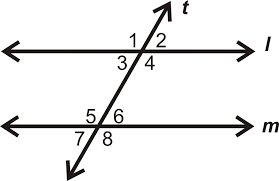• If a transversal intersects two parallel lines, then each pair of corresponding angles is equal.
(i) ∠1=∠5 (ii) ∠2=∠6
(iii) ∠3=∠7 (iv) ∠4=∠8
• If a transversal intersects two parallel lines, then each pair of alternate interior angles is equal.
(i) ∠3=∠6 (ii) ∠4=∠5
• If a transversal intersects two parallel lines, then each pair of interior angles on the same side of the transversal is supplementary.
(i) ∠3+∠5=1800 (ii) ∠4+∠6=1800

### Checking if two or more lines are parallel

• There are three conditions to check whether the two lines are parallel. They are:
(i) If a transversal intersects two lines such that a pair of corresponding angles is equal, then the two lines are parallel to each other.
(ii) If a transversal intersects two lines such that a pair of alternate interior angles is equal, then the two lines are parallel.
(iii) If a transversal intersects two lines such that a pair of interior angles on the same side of the transversal is supplementary, then the two lines are parallel.

To know more about Parallel Lines and a Transversal, visit here.

## Intersecting Lines and Pairs of Angles

### Supplementary angles

• Two angles whose sum is 1800 are called supplementary angles.
Example: 1100+700=900
∴ 1100 and 700 angles are supplementary angles.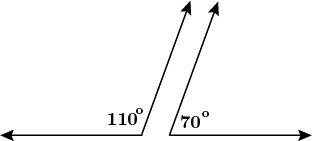To know more about Supplementary Angles, visit here.

• Two angles are adjacent, if they have
(i) A common vertex
(ii) A common arm
(iii) Their non-common arms on different sides of the common arm.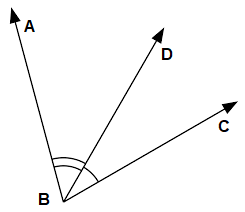Here ∠ABD and ∠DBC are adjacent angles.

### Linear Pair

• Linear pair of angles are adjacent angles whose sum is equal to 180∘.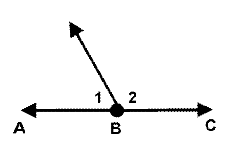Here, 1 and 2 are linear pair of angles.

### Vertically Opposite Angles

• Vertically opposite angles are formed when two straight lines intersect each other at a common point.
• Vertically opposite angles are equal.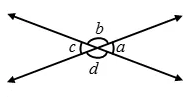Here, the following pairs of angles are vertically opposite angles.
(i) a and c
(ii) b and d

To know more about Adjacent Angle, Linear Pair and Vertically Opposite Angles, visit here.

### Intersecting and Non-Intersecting lines

• Intersecting lines are lines which intersect at a common point called the point of intersection.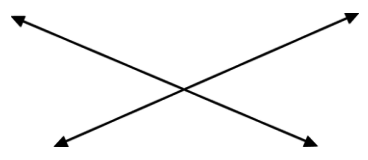• Parallel lines are lines which do not intersect at any point. Parallel lines are also known as non- intersecting lines.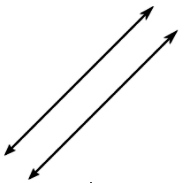To know more about Intersecting and Non-Intersecting lines, visit here.

## Basic Properties of a Triangle

#### For more information on Basic Properties of Triangle, watch the below video.### Sum of Interior Angles in a Triangle

• Angle sum property of a triangle: Sum of all interior angles of a triangle is 1800.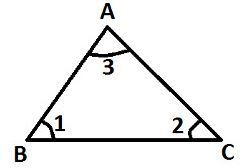In △ABC, ∠1+∠2+∠3=1800

### The exterior angle of a triangle = Sum of opposite internal angles

• If a side of a triangle is produced, then the exterior angle so formed is equal to the sum of the two interior opposite angles.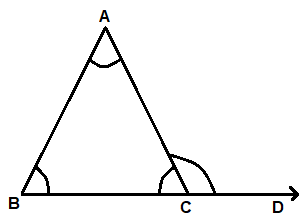In △ABC, ∠CAB+∠ABC=∠ACD.

#### For more information on Exterior Angle Theorem, watch the below video.To know more about “Basic Properties of a Triangle”, visit here.

## Frequently Asked Questions on CBSE Class 7 Maths Notes Chapter 5 Lines and Angles

Q1

### What is the definition of a ray?

In geometry, a ray is usually taken as a half-infinite line with one of the two points and. taken to be at infinity.

Q2